Open in App
Not now

# Imbalanced-Learn module in Python

• Last Updated : 11 Dec, 2020

Imbalanced-Learn is a Python module that helps in balancing the datasets which are highly skewed or biased towards some classes. Thus, it helps in resampling the classes which are otherwise oversampled or undesampled. If there is a greater imbalance ratio, the output is biased to the class which has a higher number of examples. The following dependencies need to be installed to use imbalanced-learn:

• scipy(>=0.19.1)
• numpy(>=1.13.3)
• scikit-learn(>=0.23)
• joblib(>=0.11)
• keras 2 (optional)
• tensorflow (optional)

To install imbalanced-learn just type in :

`pip install imbalanced-learn`

The resampling of data is done in 2 parts:

Estimator: It implements a fit method which is derived from scikit-learn. The data and targets are both in the form of a 2D array

`estimator = obj.fit(data, targets)`

Resampler: The fit_resample method resample the data and targets into a dictionary with a key-value pair of data_resampled and targets_resampled.

`data_resampled, targets_resampled = obj.fit_resample(data, targets)`

The Imbalanced Learn module has different algorithms for oversampling and undersampling:

We will use the built-in dataset called the make_classification dataset which return

• x: a matrix of n_samples*n_features and
• y: an array of integer labels.

Click dataset to get the dataset used.

## Python3

 `# import required modules``from` `sklearn.datasets ``import` `make_classification`` ` `# define dataset``x, y ``=` `make_classification(n_samples``=``10000``, ``                           ``weights``=``[``0.99``], ``                           ``flip_y``=``0``)``print``(``'x:\n'``, X)``print``(``'y:\n'``, y)`

Output: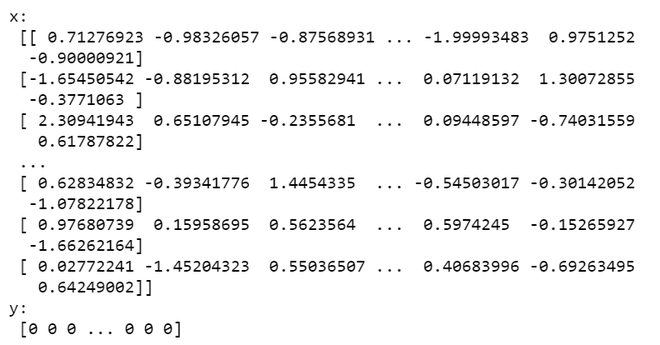Below are some programs in which depict how to apply oversampling and undersampling to the dataset:

### Oversampling

• Random Over Sampler: It is a naive method where classes that have low examples are generated and randomly resampled.

Syntax:

from imblearn.over_sampling import RandomOverSampler

Parameters(optional): sampling_strategy=’auto’, return_indices=False, random_state=None, ratio=None

Implementation:
oversample = RandomOverSampler(sampling_strategy=’minority’)
X_oversample,Y_oversample=oversample.fit_resample(X,Y)

Return Type:a matrix with the shape of n_samples*n_features

Example:

## Python3

 `# import required modules``from` `sklearn.datasets ``import` `make_classification``from` `imblearn.over_sampling ``import` `RandomOverSampler`` ` `# define dataset``x, y ``=` `make_classification(n_samples``=``10000``, ``                           ``weights``=``[``0.99``], ``                           ``flip_y``=``0``)`` ` `oversample ``=` `RandomOverSampler(sampling_strategy``=``'minority'``)``x_over, y_over ``=` `oversample.fit_resample(x, y)`` ` `# print the features and the labels``print``(``'x_over:\n'``, x_over)``print``(``'y_over:\n'``, y_over)`

Output: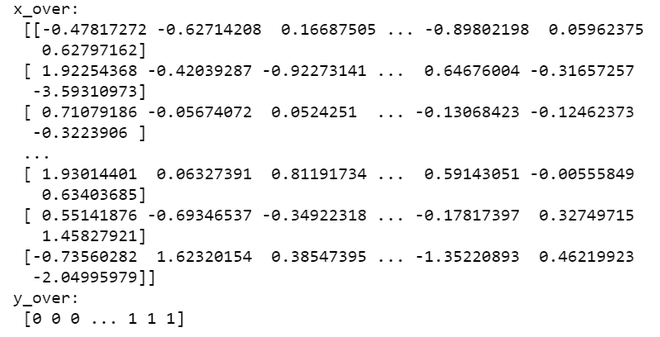•  SMOTE, ADASYN: Synthetic Minority Oversampling Technique (SMOTE)  and the Adaptive Synthetic (ADASYN) are 2 methods used in oversampling. These also generate low examples but ADASYN takes into account the density of distribution to distribute the data points evenly.

Syntax:

Parameters(optional):*, sampling_strategy=’auto’, random_state=None, n_neighbors=5, n_jobs=None

Implementation:
smote = SMOTE(ratio=’minority’)
X_smote,Y_smote=smote.fit_resample(X,Y)

Return Type:a matrix with the shape of n_samples*n_features

Example:

## Python3

 `# import required modules``from` `sklearn.datasets ``import` `make_classification``from` `imblearn.over_sampling ``import` `SMOTE`` ` `# define dataset``x, y ``=` `make_classification(n_samples``=``10000``, weights``=``[``0.99``], flip_y``=``0``)``smote ``=` `SMOTE()``x_smote, y_smote ``=` `smote.fit_resample(x, y)`` ` `# print the features and the labels``print``(``'x_smote:\n'``, x_smote)``print``(``'y_smote:\n'``, y_smote)`

Output: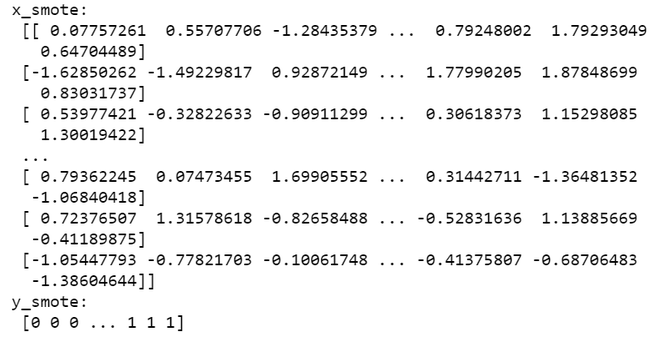### Undersampling

• Edited Nearest Neighbours: This algorithm removes any sample which has labels different from those of its adjoining classes.

Syntax:

from imblearn.under_sampling import EditedNearestNeighbours

Parameters(optional): sampling_strategy=’auto’, return_indices=False, random_state=None, n_neighbors=3, kind_sel=’all’, n_jobs=1, ratio=None

Implementation:
en = EditedNearestNeighbours()
X_en,Y_en=en.fit_resample(X, y)

Return Type:a matrix with the shape of n_samples*n_features

Example:

## Python3

 `# import required modules``from` `sklearn.datasets ``import` `make_classification``from` `imblearn.under_sampling ``import` `EditedNearestNeighbours`` ` `# define dataset``x, y ``=` `make_classification(n_samples``=``10000``, weights``=``[``0.99``], flip_y``=``0``)``en ``=` `EditedNearestNeighbours()``x_en, y_en ``=` `en.fit_resample(x, y)`` ` `# print the features and the labels``print``(``'x_en:\n'``, x_en)``print``(``'y_en:\n'``, y_en)`

Output: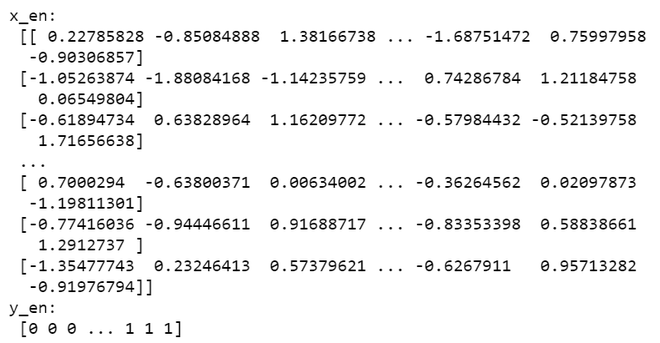• Random Under Sampler: It involves sampling any random class with or without any replacement.

Syntax:

from imblearn.under_sampling import RandomUnderSampler
Parameters(optional): sampling_strategy=’auto’, return_indices=False, random_state=None, replacement=False, ratio=None

Implementation:
undersample = RandomUnderSampler()
X_under, y_under = undersample.fit_resample(X, y)

Return Type: a matrix with the shape of n_samples*n_features

Example:

## Python3

 `# import required modules``from` `sklearn.datasets ``import` `make_classification``from` `imblearn.under_sampling ``import` `RandomUnderSampler`` ` `# define dataset``x, y ``=` `make_classification(n_samples``=``10000``, ``                           ``weights``=``[``0.99``], ``                           ``flip_y``=``0``)``undersample ``=` `RandomUnderSampler()``x_under, y_under ``=` `undersample.fit_resample(x, y)`` ` `# print the features and the labels``print``(``'x_under:\n'``, x_under)``print``(``'y_under:\n'``, y_under)`

Output: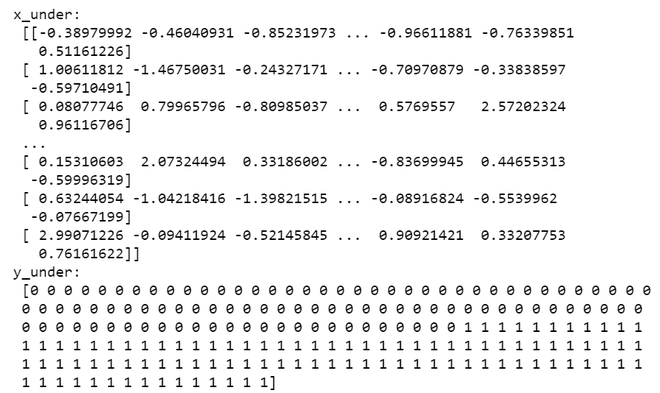My Personal Notes arrow_drop_up